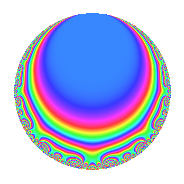Properties

 Label 8036.2.a.iLevel 8036 Weight 2 Character orbit 8036.a Self dual Yes Analytic conductor 64.168 Analytic rank 0 Dimension 4 CM No Inner twists 1

Related objects

Newspace parameters

 Level: $$N$$ = $$8036 = 2^{2} \cdot 7^{2} \cdot 41$$ Weight: $$k$$ = $$2$$ Character orbit: $$[\chi]$$ = 8036.a (trivial)

Newform invariants

 Self dual: Yes Analytic conductor: $$64.1677830643$$ Analytic rank: $$0$$ Dimension: $$4$$ Coefficient field: 4.4.25808.1 Coefficient ring: $$\Z[a_1, \ldots, a_{5}]$$ Coefficient ring index: $$1$$ Fricke sign: $$-1$$ Sato-Tate group: $\mathrm{SU}(2)$

$q$-expansion

Coefficients of the $$q$$-expansion are expressed in terms of a basis $$1,\beta_1,\beta_2,\beta_3$$ for the coefficient ring described below. We also show the integral $$q$$-expansion of the trace form.

 $$f(q)$$ $$=$$ $$q$$ $$+ ( \beta_{1} - \beta_{2} ) q^{3}$$ $$+ ( -2 + \beta_{2} - \beta_{3} ) q^{5}$$ $$+ ( 3 - \beta_{2} - \beta_{3} ) q^{9}$$ $$+O(q^{10})$$ $$q$$ $$+ ( \beta_{1} - \beta_{2} ) q^{3}$$ $$+ ( -2 + \beta_{2} - \beta_{3} ) q^{5}$$ $$+ ( 3 - \beta_{2} - \beta_{3} ) q^{9}$$ $$+ ( 1 + \beta_{1} - \beta_{2} - \beta_{3} ) q^{11}$$ $$-2 \beta_{1} q^{13}$$ $$+ ( -1 - 2 \beta_{1} + 3 \beta_{3} ) q^{15}$$ $$-2 \beta_{3} q^{17}$$ $$+ ( -2 + \beta_{1} + \beta_{2} ) q^{19}$$ $$+ ( -2 - 2 \beta_{2} ) q^{23}$$ $$+ ( 3 - 2 \beta_{1} ) q^{25}$$ $$+ ( 5 + 2 \beta_{1} - 4 \beta_{2} + \beta_{3} ) q^{27}$$ $$+ ( -2 - 2 \beta_{1} + 2 \beta_{2} ) q^{29}$$ $$+ ( 2 \beta_{2} - 2 \beta_{3} ) q^{31}$$ $$+ ( 8 + 2 \beta_{1} - 5 \beta_{2} + \beta_{3} ) q^{33}$$ $$+ ( 4 - \beta_{2} - \beta_{3} ) q^{37}$$ $$+ ( -6 + 2 \beta_{1} ) q^{39}$$ $$+ q^{41}$$ $$+ ( 2 \beta_{1} - 2 \beta_{3} ) q^{43}$$ $$+ ( -6 - 2 \beta_{1} + 7 \beta_{2} - 3 \beta_{3} ) q^{45}$$ $$+ ( 2 + 3 \beta_{1} - \beta_{2} ) q^{47}$$ $$+ ( 4 + 2 \beta_{1} - 6 \beta_{2} + 4 \beta_{3} ) q^{51}$$ $$+ ( -4 + 2 \beta_{1} - 2 \beta_{2} - 2 \beta_{3} ) q^{53}$$ $$+ ( -1 - 4 \beta_{1} + 4 \beta_{2} + \beta_{3} ) q^{55}$$ $$+ ( -4 \beta_{1} + 3 \beta_{2} + \beta_{3} ) q^{57}$$ $$+ ( -2 - 2 \beta_{2} ) q^{59}$$ $$+ ( -6 - 2 \beta_{1} ) q^{61}$$ $$+ ( -2 + 4 \beta_{1} + 2 \beta_{2} - 4 \beta_{3} ) q^{65}$$ $$+ ( 7 - \beta_{1} + \beta_{2} + \beta_{3} ) q^{67}$$ $$+ ( 6 - 2 \beta_{3} ) q^{69}$$ $$+ ( -4 + \beta_{1} + 3 \beta_{2} - 4 \beta_{3} ) q^{71}$$ $$+ ( -4 + \beta_{2} - 3 \beta_{3} ) q^{73}$$ $$+ ( -6 + 5 \beta_{1} - 3 \beta_{2} ) q^{75}$$ $$+ ( -6 - \beta_{1} + 3 \beta_{2} ) q^{79}$$ $$+ ( 7 + 6 \beta_{1} - 3 \beta_{2} - 3 \beta_{3} ) q^{81}$$ $$+ ( 2 + 4 \beta_{1} - 2 \beta_{3} ) q^{83}$$ $$+ ( 4 - 4 \beta_{1} + 6 \beta_{2} - 2 \beta_{3} ) q^{85}$$ $$+ ( -12 - 2 \beta_{1} + 4 \beta_{2} + 2 \beta_{3} ) q^{87}$$ $$+ ( 4 \beta_{1} - 2 \beta_{2} ) q^{89}$$ $$+ ( -2 - 4 \beta_{2} + 6 \beta_{3} ) q^{93}$$ $$+ ( 7 - 2 \beta_{1} - 4 \beta_{2} + 3 \beta_{3} ) q^{95}$$ $$+ ( -2 + 4 \beta_{3} ) q^{97}$$ $$+ ( 16 + 7 \beta_{1} - 7 \beta_{2} - 4 \beta_{3} ) q^{99}$$ $$+O(q^{100})$$ $$\operatorname{Tr}(f)(q)$$ $$=$$ $$4q$$ $$\mathstrut -\mathstrut 2q^{3}$$ $$\mathstrut -\mathstrut 4q^{5}$$ $$\mathstrut +\mathstrut 12q^{9}$$ $$\mathstrut +\mathstrut O(q^{10})$$ $$4q$$ $$\mathstrut -\mathstrut 2q^{3}$$ $$\mathstrut -\mathstrut 4q^{5}$$ $$\mathstrut +\mathstrut 12q^{9}$$ $$\mathstrut +\mathstrut 4q^{11}$$ $$\mathstrut -\mathstrut 10q^{15}$$ $$\mathstrut +\mathstrut 4q^{17}$$ $$\mathstrut -\mathstrut 6q^{19}$$ $$\mathstrut -\mathstrut 12q^{23}$$ $$\mathstrut +\mathstrut 12q^{25}$$ $$\mathstrut +\mathstrut 10q^{27}$$ $$\mathstrut -\mathstrut 4q^{29}$$ $$\mathstrut +\mathstrut 8q^{31}$$ $$\mathstrut +\mathstrut 20q^{33}$$ $$\mathstrut +\mathstrut 16q^{37}$$ $$\mathstrut -\mathstrut 24q^{39}$$ $$\mathstrut +\mathstrut 4q^{41}$$ $$\mathstrut +\mathstrut 4q^{43}$$ $$\mathstrut -\mathstrut 4q^{45}$$ $$\mathstrut +\mathstrut 6q^{47}$$ $$\mathstrut -\mathstrut 4q^{51}$$ $$\mathstrut -\mathstrut 16q^{53}$$ $$\mathstrut +\mathstrut 2q^{55}$$ $$\mathstrut +\mathstrut 4q^{57}$$ $$\mathstrut -\mathstrut 12q^{59}$$ $$\mathstrut -\mathstrut 24q^{61}$$ $$\mathstrut +\mathstrut 4q^{65}$$ $$\mathstrut +\mathstrut 28q^{67}$$ $$\mathstrut +\mathstrut 28q^{69}$$ $$\mathstrut -\mathstrut 2q^{71}$$ $$\mathstrut -\mathstrut 8q^{73}$$ $$\mathstrut -\mathstrut 30q^{75}$$ $$\mathstrut -\mathstrut 18q^{79}$$ $$\mathstrut +\mathstrut 28q^{81}$$ $$\mathstrut +\mathstrut 12q^{83}$$ $$\mathstrut +\mathstrut 32q^{85}$$ $$\mathstrut -\mathstrut 44q^{87}$$ $$\mathstrut -\mathstrut 4q^{89}$$ $$\mathstrut -\mathstrut 28q^{93}$$ $$\mathstrut +\mathstrut 14q^{95}$$ $$\mathstrut -\mathstrut 16q^{97}$$ $$\mathstrut +\mathstrut 58q^{99}$$ $$\mathstrut +\mathstrut O(q^{100})$$

Basis of coefficient ring in terms of a root $$\nu$$ of $$x^{4}\mathstrut -\mathstrut$$ $$10$$ $$x^{2}\mathstrut -\mathstrut$$ $$6$$ $$x\mathstrut +\mathstrut$$ $$9$$:

 $$\beta_{0}$$ $$=$$ $$1$$ $$\beta_{1}$$ $$=$$ $$\nu$$ $$\beta_{2}$$ $$=$$ $$($$$$\nu^{3} - 7 \nu - 3$$$$)/3$$ $$\beta_{3}$$ $$=$$ $$($$$$-\nu^{3} + 3 \nu^{2} + 7 \nu - 12$$$$)/3$$
 $$1$$ $$=$$ $$\beta_0$$ $$\nu$$ $$=$$ $$\beta_{1}$$ $$\nu^{2}$$ $$=$$ $$\beta_{3}\mathstrut +\mathstrut$$ $$\beta_{2}\mathstrut +\mathstrut$$ $$5$$ $$\nu^{3}$$ $$=$$ $$3$$ $$\beta_{2}\mathstrut +\mathstrut$$ $$7$$ $$\beta_{1}\mathstrut +\mathstrut$$ $$3$$

Embeddings

For each embedding $$\iota_m$$ of the coefficient field, the values $$\iota_m(a_n)$$ are shown below.

For more information on an embedded modular form you can click on its label.

Label $$\iota_m(\nu)$$ $$a_{2}$$ $$a_{3}$$ $$a_{4}$$ $$a_{5}$$ $$a_{6}$$ $$a_{7}$$ $$a_{8}$$ $$a_{9}$$ $$a_{10}$$
1.1
 −1.55466 −2.46810 3.31526 0.707500
0 −2.92968 0 3.33307 0 0 0 5.58303 0
1.2 0 −2.21551 0 −3.59669 0 0 0 1.90849 0
1.3 0 −0.0950939 0 −1.17025 0 0 0 −2.99096 0
1.4 0 3.24028 0 −2.56613 0 0 0 7.49944 0
 $$n$$: e.g. 2-40 or 990-1000 Significant digits: Format: Complex embeddings Normalized embeddings Satake parameters Satake angles

Inner twists

This newform does not admit any (nontrivial) inner twists.

Atkin-Lehner signs

$$p$$ Sign
$$2$$ $$-1$$
$$7$$ $$-1$$
$$41$$ $$-1$$

Hecke kernels

This newform can be constructed as the intersection of the kernels of the following linear operators acting on $$S_{2}^{\mathrm{new}}(\Gamma_0(8036))$$:

 $$T_{3}^{4}$$ $$\mathstrut +\mathstrut 2 T_{3}^{3}$$ $$\mathstrut -\mathstrut 10 T_{3}^{2}$$ $$\mathstrut -\mathstrut 22 T_{3}$$ $$\mathstrut -\mathstrut 2$$ $$T_{5}^{4}$$ $$\mathstrut +\mathstrut 4 T_{5}^{3}$$ $$\mathstrut -\mathstrut 8 T_{5}^{2}$$ $$\mathstrut -\mathstrut 44 T_{5}$$ $$\mathstrut -\mathstrut 36$$ $$T_{11}^{4}$$ $$\mathstrut -\mathstrut 4 T_{11}^{3}$$ $$\mathstrut -\mathstrut 18 T_{11}^{2}$$ $$\mathstrut +\mathstrut 18 T_{11}$$ $$\mathstrut +\mathstrut 54$$